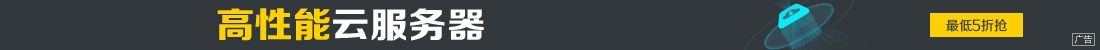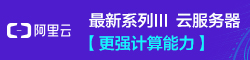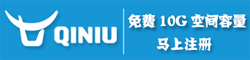# python爬虫 正则表达式使用技巧及爬取个人博客的实例讲解

••1.re模块

Python通过re模块提供对正则表达式的支持，使用正则表达式之前需要导入该库。

```import re

```

import re其基本步骤是先将正则表达式的字符串形式编译为Pattern实例，然后使用Pattern实例处理文本并获得一个匹配（Match）实例，再使用Match实例获得所需信息。常用的函数是findall，原型如下：

```findall(string[, pos[, endpos]]) | re.findall(pattern, string[, flags])
```

(1)re.I(re.IGNORECASE)：忽略大小写（括号内是完整写法）

(2)re.M(re.MULTILINE)：允许多行模式

(3)re.S(re.DOTALL)：支持点任意匹配模式

Pattern对象是一个编译好的正则表达式，通过Pattern提供的一系列方法可以对文本进行匹配查找。Pattern不能直接实例化，必须使用re.compile()进行构造。

2.complie方法

re正则表达式模块包括一些常用的操作函数，比如complie()函数。其原型如下：

```compile(pattern[,flags] )

```

```>>> import re
>>> string="A1.45，b5，6.45，8.82"
>>> regex = re.compile(r"\d+\.?\d*")
>>> print regex.findall(string)
['1.45', '5', '6.45', '8.82']
>>> ```

3.match方法

match方法是从字符串的pos下标处起开始匹配pattern，如果pattern结束时已经匹配，则返回一个Match对象；如果匹配过程中pattern无法匹配，或者匹配未结束就已到达endpos，则返回None。该方法原型如下：

```match(string[, pos[, endpos]]) | re.match(pattern, string[, flags])

```

4.search方法

search方法用于查找字符串中可以匹配成功的子串。从字符串的pos下标处起尝试匹配pattern，如果pattern结束时仍可匹配，则返回一个Match对象；若无法匹配，则将pos加1后重新尝试匹配；直到pos=endpos时仍无法匹配则返回None。 函数原型如下：

```search(string[, pos[, endpos]]) | re.search(pattern, string[, flags])

```

5.group和groups方法

group([group1, …])方法用于获得一个或多个分组截获的字符串，当它指定多个参数时将以元组形式返回。groups([default])方法以元组形式返回全部分组截获的字符串，相当于调用group(1,2,…last)。default表示没有截获字符串的组以这个值替代，默认为None。

1.抓取标签间的内容

(1) 抓取title标签间的内容

```# coding=utf-8
import re
import urllib
url = "http://www.baidu.com/"
title = re.findall(r'<title>(.*?)</title>', content)
print title
# 百度一下，你就知道```

```pat = r'(?<=<title>).*?(?=</title>)'
ex = re.compile(pat, re.M|re.S)
obj = re.search(ex, content)
title = obj.group()
print title
# 百度一下，你就知道```

(2) 抓取超链接标签间的内容

```# coding=utf-8
import re
import urllib
url = "http://www.baidu.com/"

#获取完整超链接
res = r"<a.*?href=.*?<\/a>"
urls = re.findall(res, content)
for u in urls:
print unicode(u,'utf-8')

#获取超链接<a>和</a>之间内容
res = r'<a .*?>(.*?)</a>'
texts = re.findall(res, content, re.S|re.M)
for t in texts:
print unicode(t,'utf-8')```

```#获取完整超链接
<a href="http://news.baidu.com" rel="external nofollow" rel="external nofollow" name="tj_trnews" class="mnav">新闻</a>
<a href="http://www.hao123.com" rel="external nofollow" rel="external nofollow" name="tj_trhao123" class="mnav">hao123</a>
<a href="http://map.baidu.com" rel="external nofollow" rel="external nofollow" name="tj_trmap" class="mnav">地图</a>
<a href="http://v.baidu.com" rel="external nofollow" rel="external nofollow" name="tj_trvideo" class="mnav">视频</a>
...

#获取超链接<a>和</a>之间内容

hao123

...```

(3) 抓取tr\td标签间的内容

```<html>
<body>
<table border=1>
<tr><th>学号</th><th>姓名</th></tr>
<tr><td>1001</td><td>杨秀璋</td></tr>
<tr><td>1002</td><td>严娜</td></tr>
</table>
</body>
</html>```

```# coding=utf-8
import re
import urllib

#获取<tr></tr>间内容
res = r'<tr>(.*?)</tr>'
texts = re.findall(res, content, re.S|re.M)
for m in texts:
print m

#获取<th></th>间内容
for m in texts:
res_th = r'<th>(.*?)</th>'
m_th = re.findall(res_th, m, re.S|re.M)
for t in m_th:
print t

#直接获取<td></td>间内容
res = r'<td>(.*?)</td><td>(.*?)</td>'
texts = re.findall(res, content, re.S|re.M)
for m in texts:
print m,m```

```>>>
<th>学号</th><th>姓名</th>
<td>1001</td><td>杨秀璋</td>
<td>1002</td><td>严娜</td>

1001 杨秀璋
1002 严娜
>>>```

2.抓取标签中的参数

(1) 抓取超链接标签的URL

HTML超链接的基本格式为“<a href=URL>链接内容</a>”，现在需要获取其中的URL链接地址，方法如下：

```# coding=utf-8
import re

content = '''
<a href="http://news.baidu.com" rel="external nofollow" rel="external nofollow" name="tj_trnews" class="mnav">新闻</a>
<a href="http://www.hao123.com" rel="external nofollow" rel="external nofollow" name="tj_trhao123" class="mnav">hao123</a>
<a href="http://map.baidu.com" rel="external nofollow" rel="external nofollow" name="tj_trmap" class="mnav">地图</a>
<a href="http://v.baidu.com" rel="external nofollow" rel="external nofollow" name="tj_trvideo" class="mnav">视频</a>
'''

res = r"(?<=href=\").+?(?=\")|(?<=href=\').+?(?=\')"
urls = re.findall(res, content, re.I|re.S|re.M)
for url in urls:
print url ```

```>>>
http://news.baidu.com
http://www.hao123.com
http://map.baidu.com
http://v.baidu.com
>>> ```

(2) 抓取图片超链接标签的URL

HTML插入图片使用标签的基本格式为“<img src=图片地址 />”，则需要获取图片URL链接地址的方法如下：

```content = '''<img alt="Python" src="http://www..csdn.net/eastmount.jpg" />'''
urls = re.findall('src="(.*?)"', content, re.I|re.S|re.M)
print urls
# ['http://www..csdn.net/eastmount.jpg']

```

(3) 获取URL中最后一个参数

```content = '''<img alt="Python" src="http://www..csdn.net/eastmount.jpg" />'''
urls = 'http://www..csdn.net/eastmount.jpg'
name = urls.split('/')[-1]
print name
# eastmount.jpg```

3.字符串处理及替换

```start = content.find(r'<table class="infobox"') #起点位置
end = content.find(r'</table>')    #重点点位置
infobox = text[start:end]
print infobox
```

```# coding=utf-8
import re

content = '''
<tr><td>1001</td><td>杨秀璋<br /></td></tr>
<tr><td>1002</td><td>颜&nbsp;娜</td></tr>
<tr><td>1003</td><td><B>Python</B></td></tr>
'''

res = r'<td>(.*?)</td><td>(.*?)</td>'
texts = re.findall(res, content, re.S|re.M)
for m in texts:
print m,m```

```>>>
1001 杨秀璋<br />
1002 颜 娜
1003 <B>Python</B>
>>>
```

```# coding=utf-8
import re

content = '''
<tr><td>1001</td><td>杨秀璋<br /></td></tr>
<tr><td>1002</td><td>颜&nbsp;娜</td></tr>
<tr><td>1003</td><td><B>Python</B></td></tr>
'''

res = r'<td>(.*?)</td><td>(.*?)</td>'
texts = re.findall(res, content, re.S|re.M)
for m in texts:
value0 = m.replace('<br />', '').replace('&nbsp;', '')
value1 = m.replace('<br />', '').replace('&nbsp;', '')
if '<B>' in value1:
m_value = re.findall(r'<B>(.*?)</B>', value1, re.S|re.M)
print value0, m_value
else:
print value0, value1```

```>>>
1001 杨秀璋
1002 颜娜
1003 Python
>>> ```1.博客网址的标题（title）内容。

2.爬取所有图片的超链接，比如爬取<img src=”xxx.jpg” />中的“xxx.jpg”。

3.分别爬取博客首页中的四篇文章的标题、超链接及摘要内容，比如标题为“再见北理工：忆北京研究生的编程时光”。

1.分析过程```<div class="essay">
<h1 style="text-align:center">
<a href="http://blog.csdn.net/eastmount/.../52201984" rel="external nofollow" >

</a>
</h1>
<p style="text-indent: 2em;">

</p>
</div>```

<div class=”essay2”></div>和<div class=”essay3”></div>。```import re
import urllib

url = "http://www.eastmountyxz.com/"
urls = re.findall(r'src="(.*?)"', content)
for url in urls:
print url```

```import re
import urllib
url = "http://www.eastmountyxz.com/"
start = content.find(r'<div class="essay">')
end = content.find(r'<div class="essay1">')
print content[start:end]```

(1) 调用urllib库的urlopen()函数打开博客地址，并读取内容赋值给content变量。

(2) 调用find()函数查找特定的内容，比如class属性为“essay”的div标签，依次定位获取开始和结束的位置。

(3) 进行下一步分析，获取源码中的超链接和标题等内容。

```import re
import urllib

url = "http://www.eastmountyxz.com/"
start = content.find(r'<div class="essay">')
end = content.find(r'<div class="essay1">')
page = content[start:end]

res = r"(?<=href=\").+?(?=\")|(?<=href=\').+?(?=\')"
t1 = re.findall(res, page) #超链接
print t1
t2 = re.findall(r'<a .*?>(.*?)</a>', page) #标题
print t2
t3 = re.findall('<p style=.*?>(.*?)</p>', page, re.M|re.S) #摘要(
print t3```

```>>>
http://blog.csdn.net/eastmount/article/details/52201984

两年前，我本科毕业写了这样一篇文章：《 回忆自己的大学四年得与失 》，感慨了自己在北理软院四年的所得所失；两年后，我离开了帝都，回到了贵州家乡，准备开启一段新的教师生涯，在此也写一篇文章纪念下吧！

还是那句话：这篇文章是写给自己的，希望很多年之后，回想起自己北京的六年时光，也是美好的回忆。文章可能有点长，但希望大家像读小说一样耐心品读，....
>>> ```

2.代码实现

```#coding:utf-8
import re
import urllib

url = "http://www.eastmountyxz.com/"

#爬取标题
title = re.findall(r'<title>(.*?)</title>', content)
print title

#爬取图片地址
urls = re.findall(r'src="(.*?)"', content)
for url in urls:
print url

#爬取内容
start = content.find(r'<div class="essay">')
end = content.find(r'<div class="essay1">')
page = content[start:end]
res = r"(?<=href=\").+?(?=\")|(?<=href=\').+?(?=\')"
t1 = re.findall(res, page) #超链接
print t1
t2 = re.findall(r'<a .*?>(.*?)</a>', page) #标题
print t2
t3 = re.findall('<p style=.*?>(.*?)</p>', page, re.M|re.S) #摘要(
print t3
print ''

start = content.find(r'<div class="essay1">')
end = content.find(r'<div class="essay2">')
page = content[start:end]
res = r"(?<=href=\").+?(?=\")|(?<=href=\').+?(?=\')"
t1 = re.findall(res, page) #超链接
print t1
t2 = re.findall(r'<a .*?>(.*?)</a>', page) #标题
print t2
t3 = re.findall('<p style=.*?>(.*?)</p>', page, re.M|re.S) #摘要(
print t3```